Next: MINIMUM B-VERTEX SEPARATOR Up: Cuts and Connectivity Previous: MINIMUM RATIO-CUT   Index

### MINIMUM B-BALANCED CUT

• INSTANCE: Graph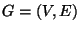, a vertex-weight function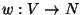, an edge-cost function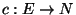, and a rational b,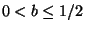.

• SOLUTION: A cut C, i.e., a subset, such thatwhere w(V') denotes the sum of the weights of the vertices in V'.

• MEASURE: The weight of the cut, i.e.,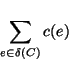where.

• Bad News: Not approximable within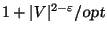for any.

• Comment: Also called Minimum b-Edge Separator. There is a polynomial algorithm that finds a b-balanced cut within an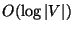factor of the optimal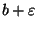-balanced cut for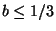. Approximable within 2 for planar graphs forif the vertex weights are polynomially bounded . Not approximable within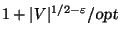for graphs of maximum degree 3 . The unweighted problem admits a PTAS if every vertex has degree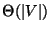for. MINIMUM K-MULTIWAY SEPARATOR, the variation in which the removal of the cut edges partitions the graph into at most k parts, where the sum of the vertex weights in each part is at most, is approximable within.Next: MINIMUM B-VERTEX SEPARATOR Up: Cuts and Connectivity Previous: MINIMUM RATIO-CUT   Index
Viggo Kann
2000-03-20